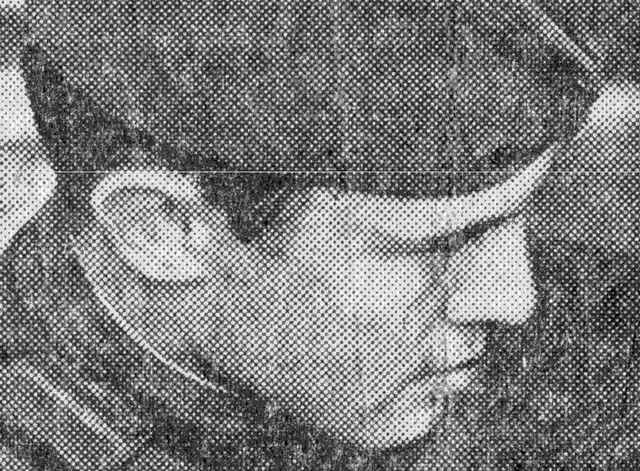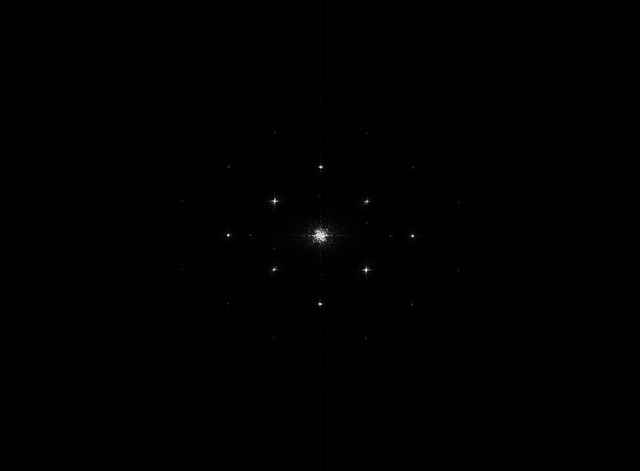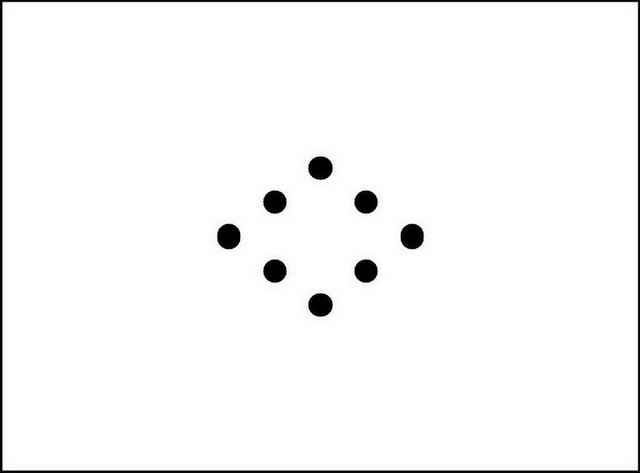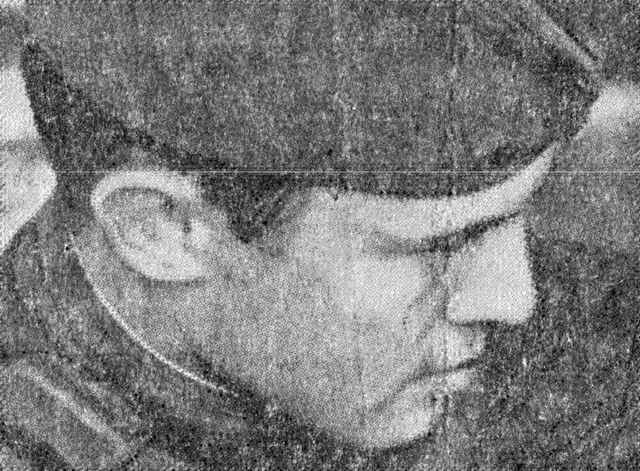OpenCV  4.0.0 Open Source Computer Vision
Periodic Noise Removing Filter

## Goal

In this tutorial you will learn:

• how to remove periodic noise in the Fourier domain

## Theory

Note
The explanation is based on the book . The image on this page is a real world image.

Periodic noise produces spikes in the Fourier domain that can often be detected by visual analysis.

### How to remove periodic noise in the Fourier domain?

Periodic noise can be reduced significantly via frequency domain filtering. On this page we use a notch reject filter with an appropriate radius to completely enclose the noise spikes in the Fourier domain. The notch filter rejects frequencies in predefined neighborhoods around a center frequency. The number of notch filters is arbitrary. The shape of the notch areas can also be arbitrary (e.g. rectangular or circular). On this page we use three circular shape notch reject filters. Power spectrum densify of an image is used for the noise spike’s visual detection.

## Source code

You can find source code in the samples/cpp/tutorial_code/ImgProc/periodic_noise_removing_filter/periodic_noise_removing_filter.cpp of the OpenCV source code library.

#include <iostream>
using namespace cv;
using namespace std;
void fftshift(const Mat& inputImg, Mat& outputImg);
void filter2DFreq(const Mat& inputImg, Mat& outputImg, const Mat& H);
void synthesizeFilterH(Mat& inputOutput_H, Point center, int radius);
void calcPSD(const Mat& inputImg, Mat& outputImg, int flag = 0);
int main()
{
if (imgIn.empty()) //check whether the image is loaded or not
{
cout << "ERROR : Image cannot be loaded..!!" << endl;
return -1;
}
imgIn.convertTo(imgIn, CV_32F);
// it needs to process even image only
Rect roi = Rect(0, 0, imgIn.cols & -2, imgIn.rows & -2);
imgIn = imgIn(roi);
// PSD calculation (start)
Mat imgPSD;
calcPSD(imgIn, imgPSD);
fftshift(imgPSD, imgPSD);
normalize(imgPSD, imgPSD, 0, 255, NORM_MINMAX);
// PSD calculation (stop)
//H calculation (start)
Mat H = Mat(roi.size(), CV_32F, Scalar(1));
const int r = 21;
synthesizeFilterH(H, Point(705, 458), r);
synthesizeFilterH(H, Point(850, 391), r);
synthesizeFilterH(H, Point(993, 325), r);
//H calculation (stop)
// filtering (start)
Mat imgOut;
fftshift(H, H);
filter2DFreq(imgIn, imgOut, H);
// filtering (stop)
imgOut.convertTo(imgOut, CV_8U);
normalize(imgOut, imgOut, 0, 255, NORM_MINMAX);
imwrite("result.jpg", imgOut);
imwrite("PSD.jpg", imgPSD);
fftshift(H, H);
normalize(H, H, 0, 255, NORM_MINMAX);
imwrite("filter.jpg", H);
return 0;
}
void fftshift(const Mat& inputImg, Mat& outputImg)
{
outputImg = inputImg.clone();
int cx = outputImg.cols / 2;
int cy = outputImg.rows / 2;
Mat q0(outputImg, Rect(0, 0, cx, cy));
Mat q1(outputImg, Rect(cx, 0, cx, cy));
Mat q2(outputImg, Rect(0, cy, cx, cy));
Mat q3(outputImg, Rect(cx, cy, cx, cy));
Mat tmp;
q0.copyTo(tmp);
q3.copyTo(q0);
tmp.copyTo(q3);
q1.copyTo(tmp);
q2.copyTo(q1);
tmp.copyTo(q2);
}
void filter2DFreq(const Mat& inputImg, Mat& outputImg, const Mat& H)
{
Mat planes = { Mat_<float>(inputImg.clone()), Mat::zeros(inputImg.size(), CV_32F) };
Mat complexI;
merge(planes, 2, complexI);
dft(complexI, complexI, DFT_SCALE);
Mat planesH = { Mat_<float>(H.clone()), Mat::zeros(H.size(), CV_32F) };
Mat complexH;
merge(planesH, 2, complexH);
Mat complexIH;
mulSpectrums(complexI, complexH, complexIH, 0);
idft(complexIH, complexIH);
split(complexIH, planes);
outputImg = planes;
}
void synthesizeFilterH(Mat& inputOutput_H, Point center, int radius)
{
Point c2 = center, c3 = center, c4 = center;
c2.y = inputOutput_H.rows - center.y;
c3.x = inputOutput_H.cols - center.x;
c4 = Point(c3.x,c2.y);
circle(inputOutput_H, center, radius, 0, -1, 8);
circle(inputOutput_H, c2, radius, 0, -1, 8);
circle(inputOutput_H, c3, radius, 0, -1, 8);
circle(inputOutput_H, c4, radius, 0, -1, 8);
}
// Function calculates PSD(Power spectrum density) by fft with two flags
// flag = 0 means to return PSD
// flag = 1 means to return log(PSD)
void calcPSD(const Mat& inputImg, Mat& outputImg, int flag)
{
Mat planes = { Mat_<float>(inputImg.clone()), Mat::zeros(inputImg.size(), CV_32F) };
Mat complexI;
merge(planes, 2, complexI);
dft(complexI, complexI);
split(complexI, planes); // planes = Re(DFT(I)), planes = Im(DFT(I))
planes.at<float>(0) = 0;
planes.at<float>(0) = 0;
// compute the PSD = sqrt(Re(DFT(I))^2 + Im(DFT(I))^2)^2
Mat imgPSD;
magnitude(planes, planes, imgPSD); //imgPSD = sqrt(Power spectrum density)
pow(imgPSD, 2, imgPSD); //it needs ^2 in order to get PSD
outputImg = imgPSD;
// logPSD = log(1 + PSD)
if (flag)
{
Mat imglogPSD;
imglogPSD = imgPSD + Scalar::all(1);
log(imglogPSD, imglogPSD);
outputImg = imglogPSD;
}
}

## Explanation

Periodic noise reduction by frequency domain filtering consists of power spectrum density calculation (for the noise spikes visual detection), notch reject filter synthesis and frequency filtering:

// it needs to process even image only
Rect roi = Rect(0, 0, imgIn.cols & -2, imgIn.rows & -2);
imgIn = imgIn(roi);
// PSD calculation (start)
Mat imgPSD;
calcPSD(imgIn, imgPSD);
fftshift(imgPSD, imgPSD);
normalize(imgPSD, imgPSD, 0, 255, NORM_MINMAX);
// PSD calculation (stop)
//H calculation (start)
Mat H = Mat(roi.size(), CV_32F, Scalar(1));
const int r = 21;
synthesizeFilterH(H, Point(705, 458), r);
synthesizeFilterH(H, Point(850, 391), r);
synthesizeFilterH(H, Point(993, 325), r);
//H calculation (stop)
// filtering (start)
Mat imgOut;
fftshift(H, H);
filter2DFreq(imgIn, imgOut, H);
// filtering (stop)

A function calcPSD() calculates power spectrum density of an image:

void calcPSD(const Mat& inputImg, Mat& outputImg, int flag)
{
Mat planes = { Mat_<float>(inputImg.clone()), Mat::zeros(inputImg.size(), CV_32F) };
Mat complexI;
merge(planes, 2, complexI);
dft(complexI, complexI);
split(complexI, planes); // planes = Re(DFT(I)), planes = Im(DFT(I))
planes.at<float>(0) = 0;
planes.at<float>(0) = 0;
// compute the PSD = sqrt(Re(DFT(I))^2 + Im(DFT(I))^2)^2
Mat imgPSD;
magnitude(planes, planes, imgPSD); //imgPSD = sqrt(Power spectrum density)
pow(imgPSD, 2, imgPSD); //it needs ^2 in order to get PSD
outputImg = imgPSD;
// logPSD = log(1 + PSD)
if (flag)
{
Mat imglogPSD;
imglogPSD = imgPSD + Scalar::all(1);
log(imglogPSD, imglogPSD);
outputImg = imglogPSD;
}
}

A function synthesizeFilterH() forms a transfer function of an ideal circular shape notch reject filter according to a center frequency and a radius:

void synthesizeFilterH(Mat& inputOutput_H, Point center, int radius)
{
Point c2 = center, c3 = center, c4 = center;
c2.y = inputOutput_H.rows - center.y;
c3.x = inputOutput_H.cols - center.x;
c4 = Point(c3.x,c2.y);
circle(inputOutput_H, center, radius, 0, -1, 8);
circle(inputOutput_H, c2, radius, 0, -1, 8);
circle(inputOutput_H, c3, radius, 0, -1, 8);
circle(inputOutput_H, c4, radius, 0, -1, 8);
}

A function filter2DFreq() filters an image in the frequency domain. The functions fftshift() and filter2DFreq() are copied from the tutorial Out-of-focus Deblur Filter.

## Result

The figure below shows an image heavily corrupted by periodical noise of various frequencies.Image corrupted by periodic noise

The noise components are easily seen as bright dots (spikes) in the Power spectrum density shown in the figure below.Power spectrum density showing periodic noise

The figure below shows a notch reject filter with an appropriate radius to completely enclose the noise spikes.Notch reject filter

The result of processing the image with the notch reject filter is shown below.Result of filtering

The improvement is quite evident. This image contains significantly less visible periodic noise than the original image.

You can also find a quick video demonstration of this filtering idea on YouTube.